# 1 Overview

In this document, we show how to conduct a differential expression (DE) analysis that controls for “unwanted variation”, e.g., batch, library preparation, and other nuisance effects, using the between-sample normalization methods proposed in Risso et al. (2014). We call this approach RUVSeq for remove unwanted variation from RNA-Seq data.

Briefly, RUVSeq works as follows. For $$n$$ samples and $$J$$ genes, consider the following generalized linear model (GLM), where the RNA-Seq read counts are regressed on both the known covariates of interest and unknown factors of unwanted variation,

$\begin{equation} \log E[Y | W, X, O] = W \alpha + X \beta + O. \end{equation}$

Here, $$Y$$ is the $$n \times J$$ matrix of observed gene-level read counts, $$W$$ is an $$n \times k$$ matrix corresponding to the factors of “unwanted variation” and $$\alpha$$ its associated $$k \times J$$ matrix of nuisance parameters, $$X$$ is an $$n \times p$$ matrix corresponding to the $$p$$ covariates of interest/factors of “wanted variation” (e.g., treatment effect) and $$\beta$$ its associated $$p \times J$$ matrix of parameters of interest, and $$O$$ is an $$n \times J$$ matrix of offsets that can either be set to zero or estimated with some other normalization procedure (such as upper-quartile normalization).

The matrix $$X$$ is a random variable, assumed to be known a priori. For instance, in the usual two-class comparison setting (e.g., treated vs. control samples), $$X$$ is an $$n \times 2$$ design matrix with a column of ones corresponding to an intercept and a column of indicator variables for the class of each sample (e.g., 0 for control and 1 for treated) (McCullagh and Nelder 1989). The matrix $$W$$ is an unobserved random variable and $$\alpha$$, $$\beta$$, and $$k$$ are unknown parameters.

The simultaneous estimation of $$W$$, $$\alpha$$, $$\beta$$, and $$k$$ is infeasible. For a given $$k$$, we consider instead the following three approaches to estimate the factors of unwanted variation $$W$$:

• RUVg uses negative control genes, assumed to have constant expression across samples;
• RUVs uses centered (technical) replicate/negative control samples for which the covariates of interest are constant;
• RUVr uses residuals, e.g., from a first-pass GLM regression of the counts on the covariates of interest.

The resulting estimate of $$W$$ can then be plugged into Equation , for the full set of genes and samples, and $$\alpha$$ and $$\beta$$ estimated by GLM regression. Normalized read counts can be obtained as residuals from ordinary least squares (OLS) regression of $$\log Y - O$$ on the estimated $$W$$.

Note that although here we illustrate the RUV approach using the GLM implementation of edgeR and DESeq2, all three RUV versions can be readily adapted to work with any DE method formulated within a GLM framework.

See Risso et al. (2014) for full details and algorithms for each of the three RUV procedures.

# 2 A typical differential expression analysis workflow

In this section, we consider the RUVg function to estimate the factors of unwanted variation using control genes. See the Sections below for examples using the RUVs and RUVr approaches.

We consider the zebrafish dataset of Ferreira et al. (2014), available through the Bioconductor package zebrafishRNASeq. The data correspond to RNA libraries for three pairs of gallein-treated and control embryonic zebrafish cell pools. For each of the 6 samples, we have RNA-Seq read counts for $$32{,}469$$ Ensembl genes and $$92$$ ERCC spike-in sequences. See Risso et al. (2014) and the zebrafishRNASeq package vignette for details.

library(RUVSeq)
library(zebrafishRNASeq)
data(zfGenes)
#>                    Ctl1 Ctl3 Ctl5 Trt9 Trt11 Trt13
#> ENSDARG00000000001  304  129  339  102    16   617
#> ENSDARG00000000002  605  637  406   82   230  1245
#> ENSDARG00000000018  391  235  217  554   451   565
#> ENSDARG00000000019 2979 4729 7002 7309  9395  3349
#> ENSDARG00000000068   89  356   41  149    45    44
#> ENSDARG00000000069  312  184  844  269   513   243
tail(zfGenes)
#>             Ctl1 Ctl3 Ctl5  Trt9 Trt11 Trt13
#> ERCC-00163   204   59  183   152   104    59
#> ERCC-00164     6    1   74    11   206    21
#> ERCC-00165   140  119   93   331    52    38
#> ERCC-00168     0    0    0     0     2     0
#> ERCC-00170   216  145  111   456   196   552
#> ERCC-00171 12869 6682 7675 47488 24322 26112

## 2.1 Filtering and exploratory data analysis

We filter out non-expressed genes, by requiring more than 5 reads in at least two samples for each gene.

filter <- apply(zfGenes, 1, function(x) length(x[x>5])>=2)
filtered <- zfGenes[filter,]
genes <- rownames(filtered)[grep("^ENS", rownames(filtered))]
spikes <- rownames(filtered)[grep("^ERCC", rownames(filtered))]

After the filtering, we are left with 20806 genes and 59 spike-ins.

We store the data in an object of S4 class SeqExpressionSet from the EDASeq package. This allows us to make full use of the plotting and normalization functionality of EDASeq. Note, however, that all the methods in RUVSeq are implemented for both SeqExpressionSet and matrix objects. See the help pages for details.

x <- as.factor(rep(c("Ctl", "Trt"), each=3))
set <- newSeqExpressionSet(as.matrix(filtered),
phenoData = data.frame(x, row.names=colnames(filtered)))
set
#> SeqExpressionSet (storageMode: lockedEnvironment)
#> assayData: 20865 features, 6 samples
#>   element names: counts, normalizedCounts, offset
#> protocolData: none
#> phenoData
#>   sampleNames: Ctl1 Ctl3 ... Trt13 (6 total)
#>   varLabels: x
#> featureData: none
#> experimentData: use 'experimentData(object)'
#> Annotation:

The boxplots of relative log expression (RLE = log-ratio of read count to median read count across sample) and plots of principal components (PC) reveal a clear need for betwen-sample normalization.

library(RColorBrewer)
colors <- brewer.pal(3, "Set2")
plotRLE(set, outline=FALSE, ylim=c(-4, 4), col=colors[x])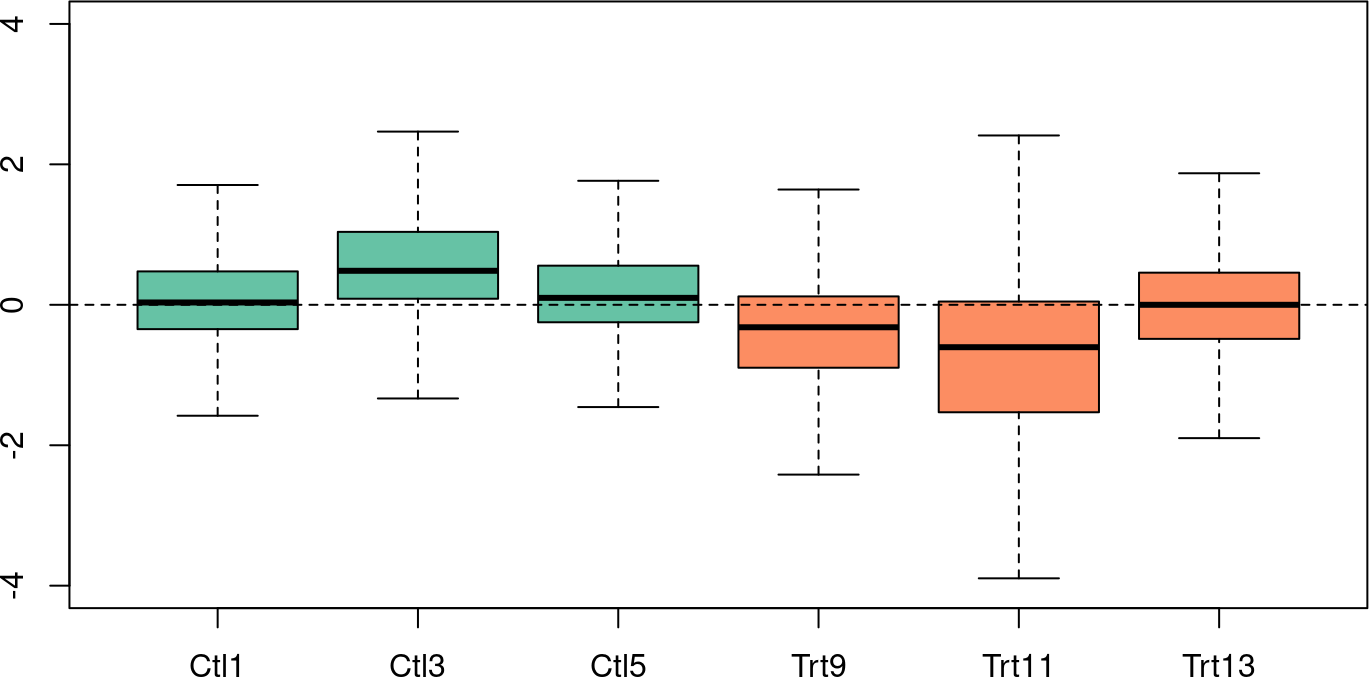plotPCA(set, col=colors[x], cex=1.2)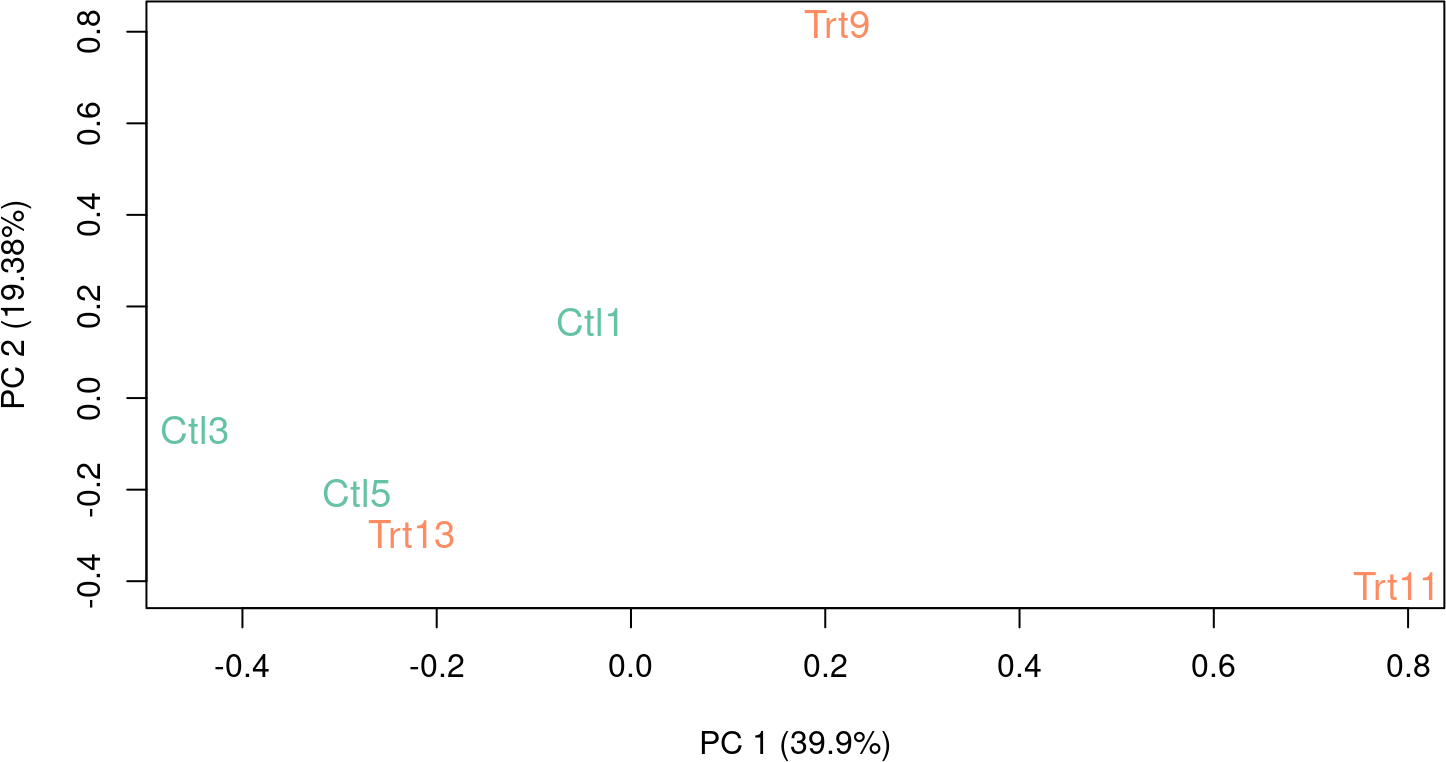We can use the betweenLaneNormalization function of EDASeq to normalize the data using upper-quartile (UQ) normalization (Bullard et al. 2010).

set <- betweenLaneNormalization(set, which="upper")
plotRLE(set, outline=FALSE, ylim=c(-4, 4), col=colors[x])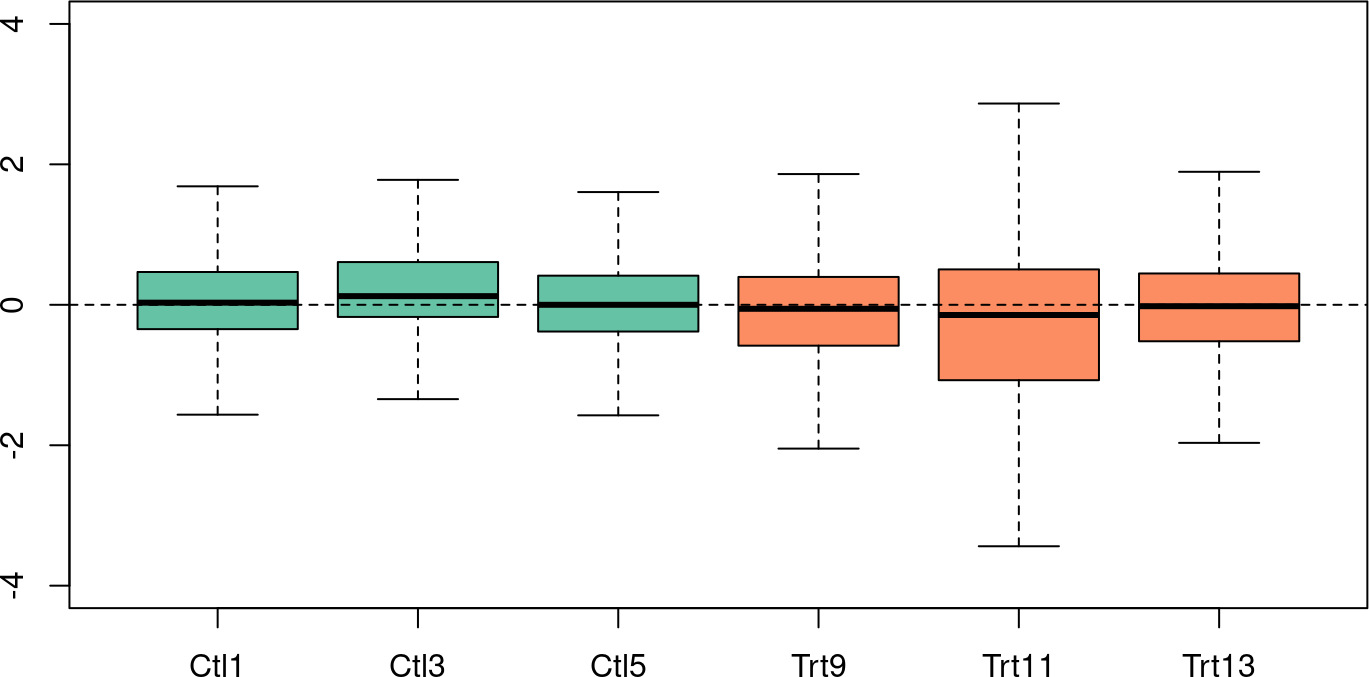plotPCA(set, col=colors[x], cex=1.2)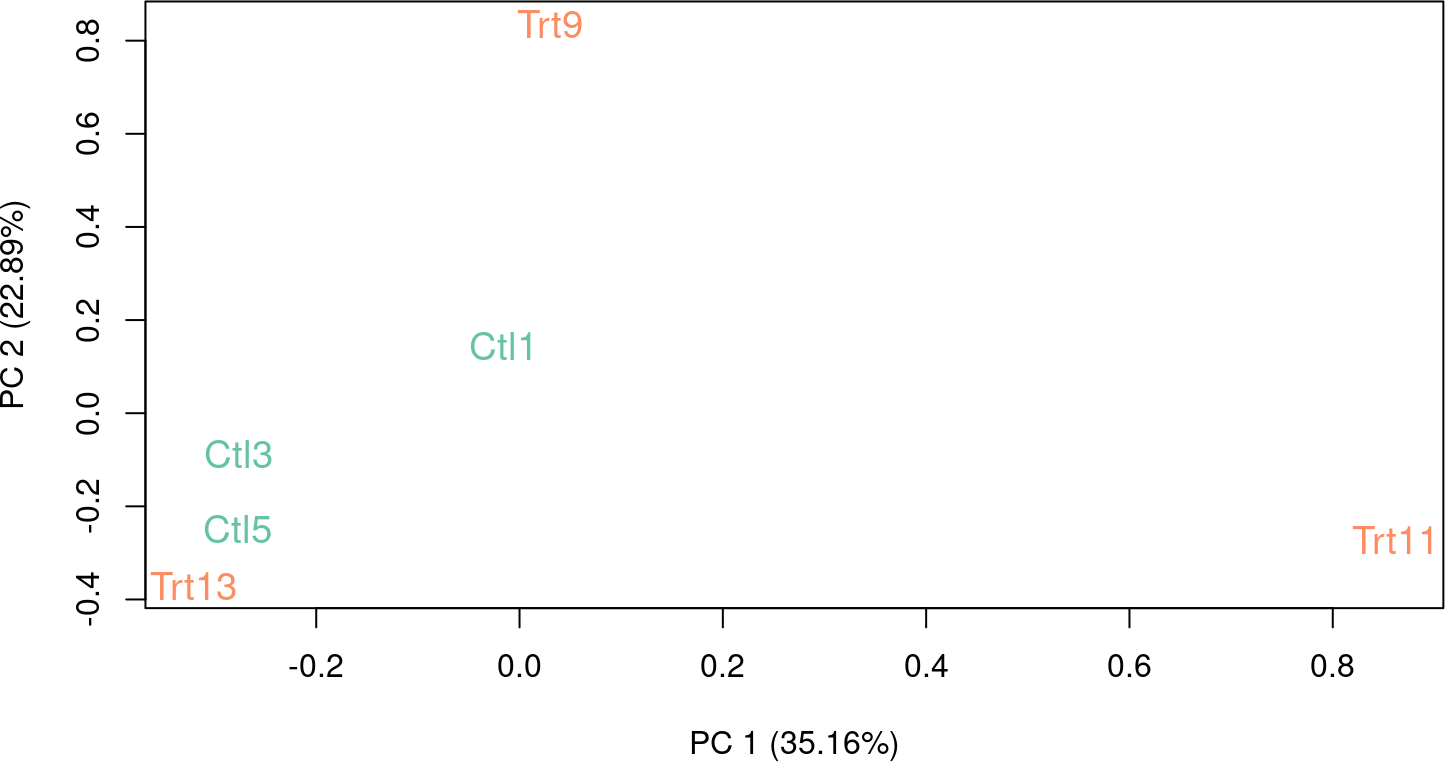After upper-quartile normalization, treated sample Trt11 still shows extra variability when compared to the rest of the samples. This is reflected by the first principal component, that is driven by the difference between Trt11 and the other samples.

## 2.2 RUVg: Estimating the factors of unwanted variation using control genes

To estimate the factors of unwanted variation, we need a set of negative control genes, i.e., genes that can be assumed not to be influenced by the covariates of interest (in the case of the zebrafish dataset, the Gallein treatment). In many cases, such a set can be identified, e.g., housekeeping genes or spike-in controls. If a good set of negative controls is not readily available, one can define a set of “in-silico empirical” controls.

Here, we use the ERCC spike-ins as controls and we consider $$k=1$$ factors of unwanted variation. See Risso et al. (2014) and Gagnon-Bartsch and Speed (2012) for a discussion on the choice of $$k$$.

set1 <- RUVg(set, spikes, k=1)
pData(set1)
#>         x         W_1
#> Ctl1  Ctl -0.04539413
#> Ctl3  Ctl  0.50347642
#> Ctl5  Ctl  0.40575319
#> Trt9  Trt -0.30773479
#> Trt11 Trt -0.68455406
#> Trt13 Trt  0.12845337
plotRLE(set1, outline=FALSE, ylim=c(-4, 4), col=colors[x])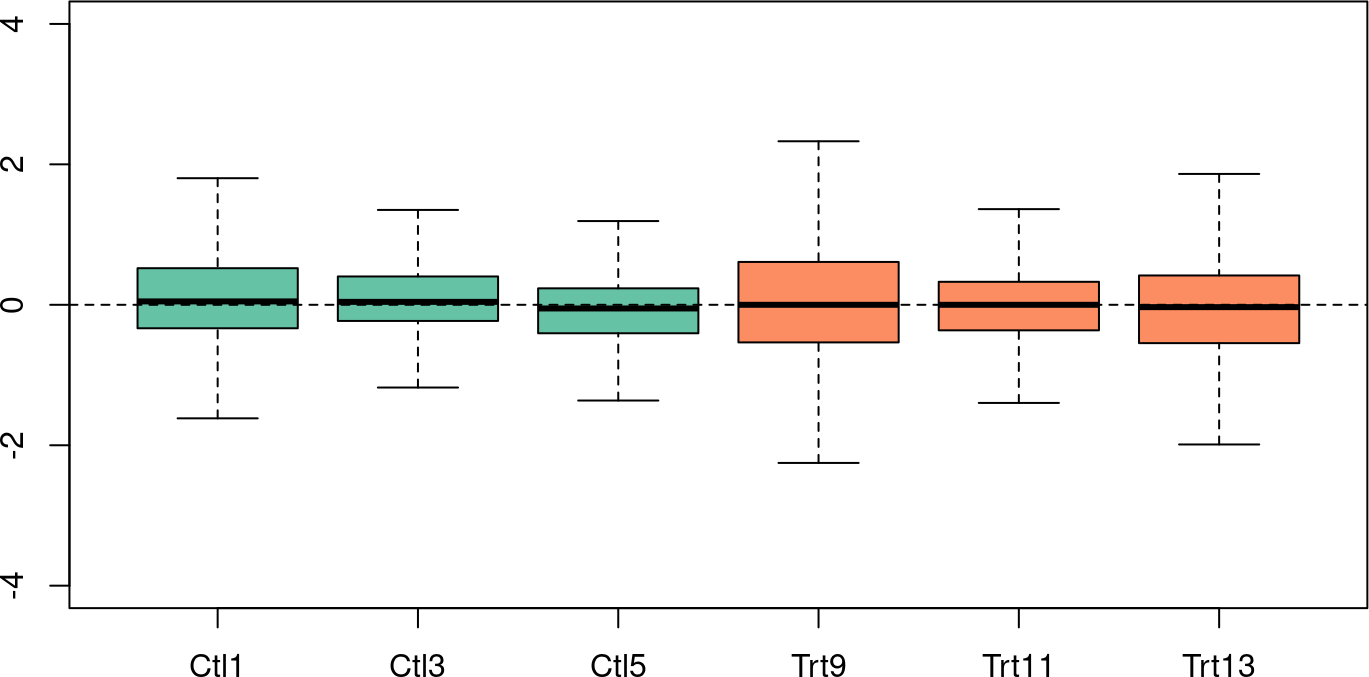plotPCA(set1, col=colors[x], cex=1.2)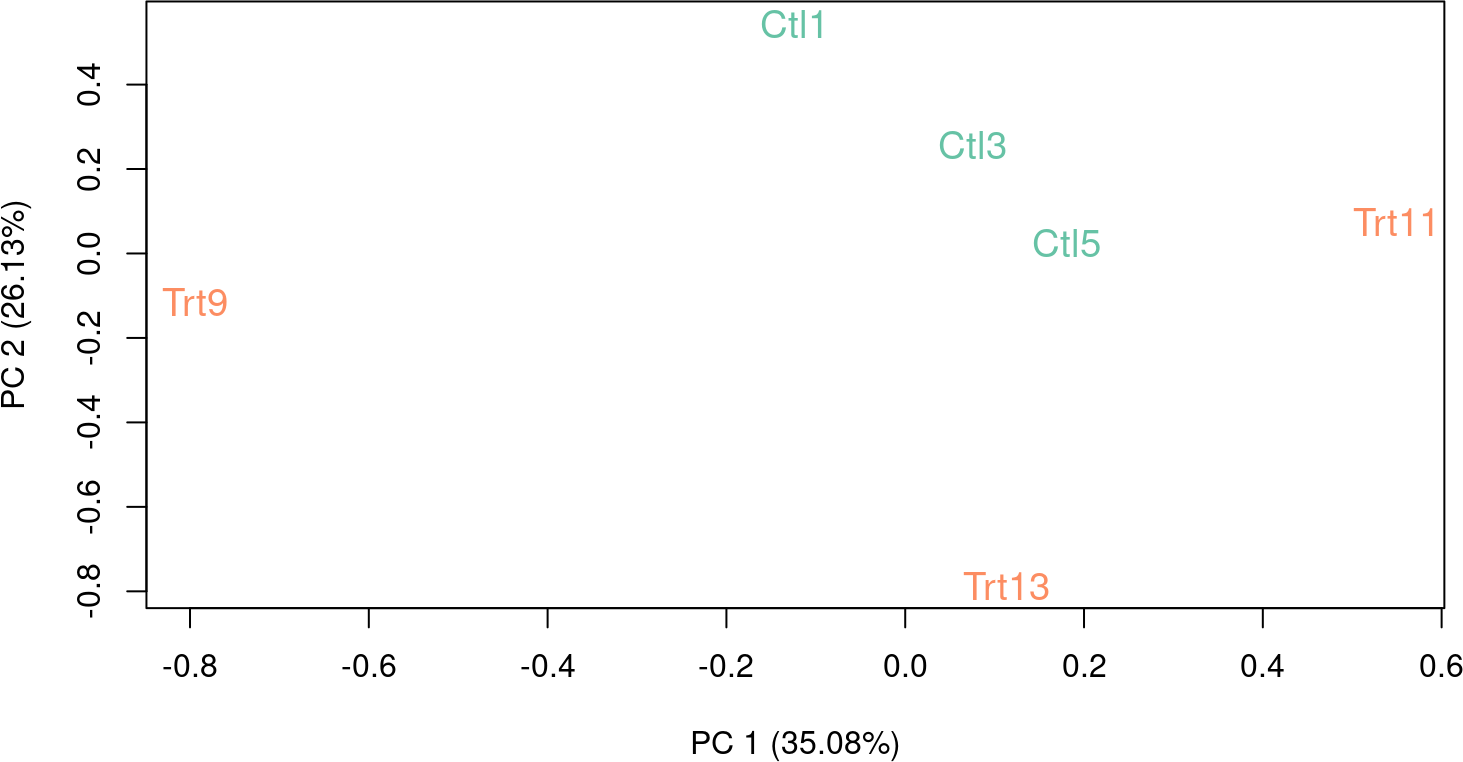The RUVg function returns two pieces of information: the estimated factors of unwanted variation (added as columns to the phenoData slot of set) and the normalized counts obtained by regressing the original counts on the unwanted factors. The normalized values are stored in the normalizedCounts slot of set and can be accessed with the normCounts method. These counts should be used only for exploration. It is important that subsequent DE analysis be done on the original counts (accessible through the counts method), as removing the unwanted factors from the counts can also remove part of a factor of interest (Gagnon-Bartsch, Jacob, and Speed 2013).

Note that one can relax the negative control gene assumption by requiring instead the identification of a set of positive or negative controls, with a priori known expression fold-changes between samples, i.e., known $$\beta$$.
One can then use the centered counts for these genes ($$\log Y - X\beta$$) for normalization purposes.

## 2.3 Differential expression analysis

Now, we are ready to look for differentially expressed genes, using the negative binomial GLM approach implemented in edgeR (see the edgeR package vignette for details). This is done by considering a design matrix that includes both the covariates of interest (here, the treatment status) and the factors of unwanted variation.

design <- model.matrix(~x + W_1, data=pData(set1))
y <- DGEList(counts=counts(set1), group=x)
y <- calcNormFactors(y, method="upperquartile")
y <- estimateGLMCommonDisp(y, design)
y <- estimateGLMTagwiseDisp(y, design)

fit <- glmFit(y, design)
lrt <- glmLRT(fit, coef=2)
topTags(lrt)
#> Coefficient:  xTrt
#>                         logFC     logCPM       LR       PValue          FDR
#> ENSDARG00000000906 -13.139608  1.3700668 41.83052 9.953755e-11 2.076851e-06
#> ENSDARG00000086720  12.005547  0.6073574 37.82485 7.738980e-10 6.212838e-06
#> ENSDARG00000040816 -15.428901 -0.3395098 37.28917 1.018489e-09 6.212838e-06
#> ENSDARG00000052057  -8.753811  6.0407525 36.98395 1.191054e-09 6.212838e-06
#> ENSDARG00000026651   9.170089  3.2087681 32.92258 9.590304e-09 4.002034e-05
#> ENSDARG00000091974 -11.869688  1.5354803 32.09091 1.471239e-08 5.116235e-05
#> ENSDARG00000094510   8.992629  1.6823082 30.89969 2.717146e-08 8.099035e-05
#> ENSDARG00000069293 -10.447662  0.2042986 30.47915 3.374746e-08 8.801759e-05
#> ENSDARG00000088842   9.993295  0.9907429 30.01999 4.276157e-08 9.913557e-05
#> ENSDARG00000041516   7.747856  7.8305044 28.67513 8.559599e-08 1.785960e-04

## 2.4 Empirical control genes

If no genes are known a priori not to be influenced by the covariates of interest, one can obtain a set of “in-silico empirical” negative controls, e.g., least significantly DE genes based on a first-pass DE analysis performed prior to RUVg normalization.

design <- model.matrix(~x, data=pData(set))
y <- DGEList(counts=counts(set), group=x)
y <- calcNormFactors(y, method="upperquartile")
y <- estimateGLMCommonDisp(y, design)
y <- estimateGLMTagwiseDisp(y, design)

fit <- glmFit(y, design)
lrt <- glmLRT(fit, coef=2)

top <- topTags(lrt, n=nrow(set))\$table
empirical <- rownames(set)[which(!(rownames(set) %in% rownames(top)[1:5000]))]

Here, we consider all but the top $$5{,}000$$ genes as ranked by edgeR $$p$$-values.

set2 <- RUVg(set, empirical, k=1)
pData(set2)
#>         x         W_1
#> Ctl1  Ctl -0.10879677
#> Ctl3  Ctl  0.23066424
#> Ctl5  Ctl  0.19926266
#> Trt9  Trt  0.07672121
#> Trt11 Trt -0.83540924
#> Trt13 Trt  0.43755790
plotRLE(set2, outline=FALSE, ylim=c(-4, 4), col=colors[x])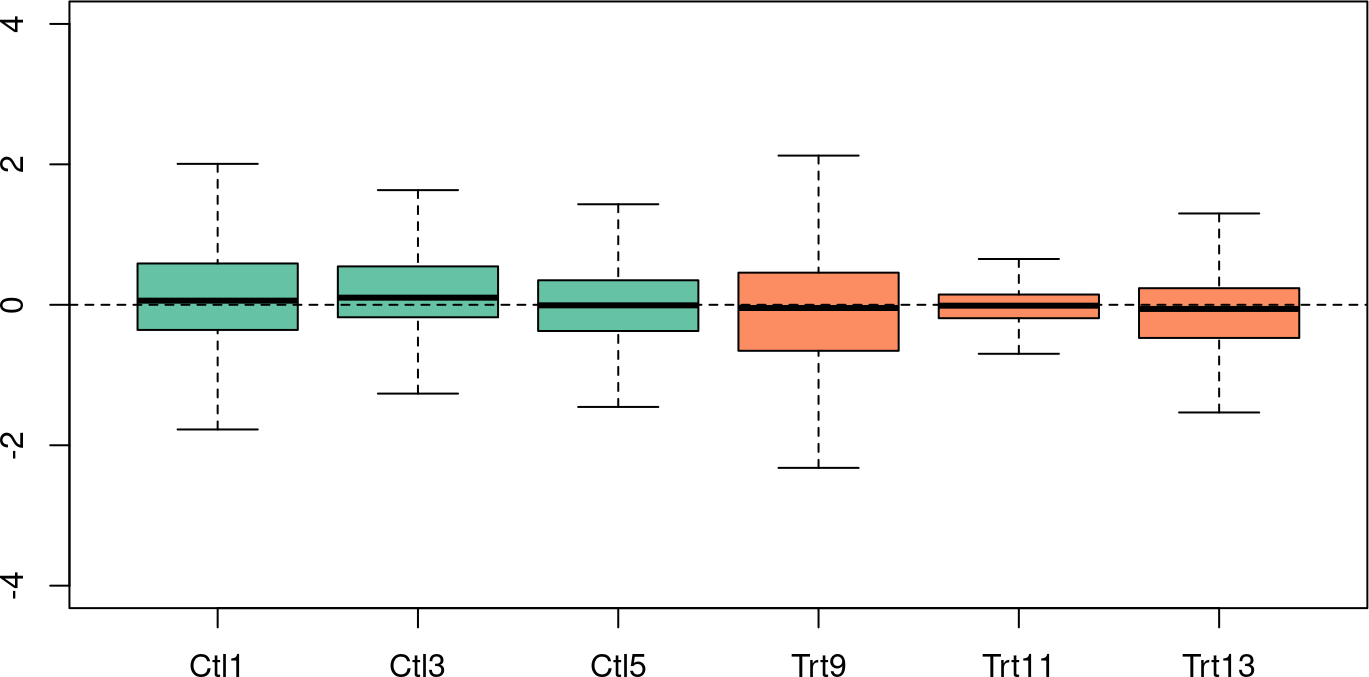plotPCA(set2, col=colors[x], cex=1.2)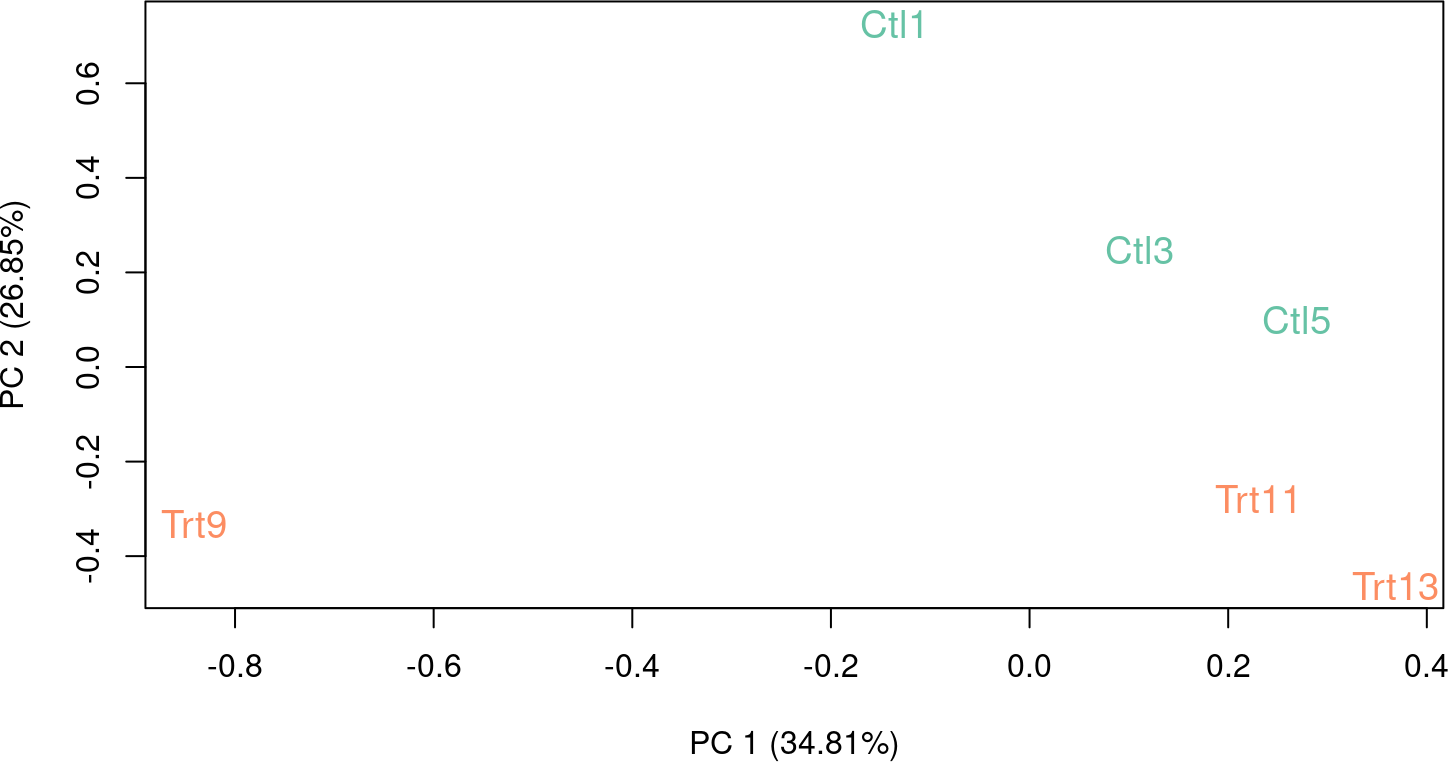## 2.5 Differential expression analysis with DESeq2

In alternative to edgeR, one can perform differential expression analysis with DESeq2. The approach is very similar, namely, we will use the same design matrix, but we need to specify it within the DESeqDataSet object.

library(DESeq2)
dds <- DESeqDataSetFromMatrix(countData = counts(set1),
colData = pData(set1),
design = ~ W_1 + x)
dds <- DESeq(dds)
#> estimating size factors
#> estimating dispersions
#> gene-wise dispersion estimates
#> mean-dispersion relationship
#> final dispersion estimates
#> fitting model and testing
res <- results(dds)
res
#> log2 fold change (MLE): x Trt vs Ctl
#> Wald test p-value: x Trt vs Ctl
#> DataFrame with 20865 rows and 6 columns
#>                     baseMean log2FoldChange     lfcSE       stat    pvalue
#>                    <numeric>      <numeric> <numeric>  <numeric> <numeric>
#> ENSDARG00000000001   232.866      0.8786748  1.389381  0.6324219  0.527111
#> ENSDARG00000000002   499.660      0.8482861  1.250605  0.6783007  0.497581
#> ENSDARG00000000018   467.170      0.9994368  0.926668  1.0785271  0.280799
#> ENSDARG00000000019  6959.358      0.0483696  1.061977  0.0455468  0.963671
#> ENSDARG00000000068   108.518      0.1188108  1.411262  0.0841876  0.932907
#> ...                      ...            ...       ...        ...       ...
#> ERCC-00163           137.567     -1.0762878  1.189237 -0.9050240 0.3654527
#> ERCC-00164            80.339     -1.1169190  2.035970 -0.5485930 0.5832848
#> ERCC-00165           142.686      0.0418648  1.385185  0.0302233 0.9758890
#> ERCC-00170           314.887      1.8354418  1.027683  1.7859999 0.0740993
#> ERCC-00171         26113.478      1.7594758  0.943496  1.8648473 0.0622028
#>                    <numeric>
#> ENSDARG00000000001  0.902319
#> ENSDARG00000000002  0.891360
#> ENSDARG00000000018  0.768956
#> ENSDARG00000000019  0.996814
#> ENSDARG00000000068  0.992634
#> ...                      ...
#> ERCC-00163          0.830022
#> ERCC-00164          0.920096
#> ERCC-00165          0.998801
#> ERCC-00170          0.495916
#> ERCC-00171          0.462520

Note that this will perform by default a Wald test of significance of the last variable in the design formula, in this case $$x$$. If one wants to perform a likelihood ratio test, she needs to specify a reduced model that includes $$W$$ (see the DESeq2 vignette for more details on the test statistics).

dds <- DESeq(dds, test="LRT", reduced=as.formula("~ W_1"))
res <- results(dds)

# 3 RUVs: Estimating the factors of unwanted variation using replicate samples}

As an alternative approach, one can use the RUVs method to estimate the factors of unwanted variation using replicate/negative control samples for which the covariates of interest are constant.

First, we need to construct a matrix specifying the replicates. In the case of the zebrafish dataset, we can consider the three treated and the three control samples as replicate groups. The function makeGroups can be used.

differences <- makeGroups(x)
differences
#>      [,1] [,2] [,3]
#> [1,]    1    2    3
#> [2,]    4    5    6

Although in principle one still needs control genes for the estimation of the factors of unwanted variation, we found that RUVs is robust to that choice and that using all the genes works well in practice (Risso et al. 2014).

set3 <- RUVs(set, genes, k=1, differences)
pData(set3)
#>         x        W_1
#> Ctl1  Ctl  0.1860825
#> Ctl3  Ctl  0.4917394
#> Ctl5  Ctl  0.4926805
#> Trt9  Trt  0.4279274
#> Trt11 Trt -0.5784460
#> Trt13 Trt  0.7289145

# 4 RUVr: Estimating the factors of unwanted variation using residuals

Finally, a third approach is to consider the residuals (e.g., deviance residuals) from a first-pass GLM regression of the counts on the covariates of interest. This can be achieved with the RUVr method.

First, we need to compute the residuals from the GLM fit, without RUVg normalization, but possibly after normalization using a method such as upper-quartile normalization.

design <- model.matrix(~x, data=pData(set))
y <- DGEList(counts=counts(set), group=x)
y <- calcNormFactors(y, method="upperquartile")
y <- estimateGLMCommonDisp(y, design)
y <- estimateGLMTagwiseDisp(y, design)

fit <- glmFit(y, design)
res <- residuals(fit, type="deviance")

Again, we can use all the genes to estimate the factors of unwanted variation.

set4 <- RUVr(set, genes, k=1, res)

# 5 Session info

sessionInfo()
#> R Under development (unstable) (2022-10-25 r83175)
#> Platform: x86_64-pc-linux-gnu (64-bit)
#> Running under: Ubuntu 22.04.1 LTS
#>
#> Matrix products: default
#> BLAS:   /home/biocbuild/bbs-3.17-bioc/R/lib/libRblas.so
#> LAPACK: /usr/lib/x86_64-linux-gnu/lapack/liblapack.so.3.10.0
#>
#> locale:
#>   LC_CTYPE=en_US.UTF-8       LC_NUMERIC=C
#>   LC_TIME=en_GB              LC_COLLATE=C
#>   LC_MONETARY=en_US.UTF-8    LC_MESSAGES=en_US.UTF-8
#>   LC_PAPER=en_US.UTF-8       LC_NAME=C
#>  LC_MEASUREMENT=en_US.UTF-8 LC_IDENTIFICATION=C
#>
#> attached base packages:
#>  stats4    stats     graphics  grDevices utils     datasets  methods
#>  base
#>
#> other attached packages:
#>   DESeq2_1.39.2               RColorBrewer_1.1-3
#>   zebrafishRNASeq_1.19.0      RUVSeq_1.33.1
#>   edgeR_3.41.1                limma_3.55.0
#>   GenomicAlignments_1.35.0    SummarizedExperiment_1.29.1
#>  MatrixGenerics_1.11.0       matrixStats_0.63.0
#>  Rsamtools_2.15.0            GenomicRanges_1.51.1
#>  Biostrings_2.67.0           GenomeInfoDb_1.35.5
#>  XVector_0.39.0              IRanges_2.33.0
#>  S4Vectors_0.37.0            BiocParallel_1.33.5
#>  Biobase_2.59.0              BiocGenerics_0.45.0
#>  BiocStyle_2.27.0
#>
#> loaded via a namespace (and not attached):
#>   DBI_1.1.3              bitops_1.0-7           deldir_1.0-6
#>   biomaRt_2.55.0         rlang_1.0.6            magrittr_2.0.3
#>   compiler_4.3.0         RSQLite_2.2.18         GenomicFeatures_1.51.2
#>  png_0.1-7              vctrs_0.5.1            stringr_1.4.1
#>  pkgconfig_2.0.3        crayon_1.5.2           fastmap_1.1.0
#>  magick_2.7.3           dbplyr_2.2.1           ellipsis_0.3.2
#>  utf8_1.2.2             rmarkdown_2.18         bit_4.0.5
#>  xfun_0.35              zlibbioc_1.45.0        cachem_1.0.6
#>  jsonlite_1.8.3         progress_1.2.2         blob_1.2.3
#>  highr_0.9              DelayedArray_0.25.0    jpeg_0.1-9
#>  parallel_4.3.0         prettyunits_1.1.1      R6_2.5.1
#>  bslib_0.4.1            stringi_1.7.8          rtracklayer_1.59.0
#>  jquerylib_0.1.4        Rcpp_1.0.9             bookdown_0.30
#>  assertthat_0.2.1       knitr_1.41             R.utils_2.12.2
#>  Matrix_1.5-3           tidyselect_1.2.0       yaml_2.3.6
#>  codetools_0.2-18       hwriter_1.3.2.1        curl_4.3.3
#>  lattice_0.20-45        tibble_3.1.8           KEGGREST_1.39.0
#>  evaluate_0.18          BiocFileCache_2.7.0    xml2_1.3.3
#>  pillar_1.8.1           BiocManager_1.30.19    filelock_1.0.2
#>  generics_0.1.3         RCurl_1.98-1.9         ggplot2_3.4.0
#>  hms_1.1.2              munsell_0.5.0          scales_1.2.1
#>  xtable_1.8-4           glue_1.6.2             tools_4.3.0
#>  interp_1.1-3           BiocIO_1.9.0           annotate_1.77.0
#>  locfit_1.5-9.6         XML_3.99-0.12          grid_4.3.0
#>  latticeExtra_0.6-30    colorspace_2.0-3       AnnotationDbi_1.61.0
#>  GenomeInfoDbData_1.2.9 restfulr_0.0.15        cli_3.4.1
#>  rappdirs_0.3.3         fansi_1.0.3            dplyr_1.0.10
#>  gtable_0.3.1           R.methodsS3_1.8.2      sass_0.4.3
#>  digest_0.6.30          aroma.light_3.29.0     geneplotter_1.77.0
#>  rjson_0.2.21           memoise_2.0.1          htmltools_0.5.3
#>  R.oo_1.25.0            lifecycle_1.0.3        httr_1.4.4
#>  bit64_4.0.5            MASS_7.3-58.1